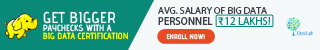Quantitative Analysis 1 - Five Number Summary

# Quantitative Analysis 1 – Five Number Summary

To be a successful analyst or be a part of great analytics team, there are 3 important dimensions one would aspire to be or have. They are technical, business and tools. Hence, we would begin with one of the sub dimension of the technical skills, i.e. being quantified self or developing quantitative skills.

As per the Informs, the definition of Analytics shall be:

“Analytics is defined as the scientific process of transforming data into insight for making better decisions.”

Analytics is quantitative in nature. Statistics and Mathematics play a major role in bringing insights from the data. Statistics and Mathematics provides an analyst with some effective tools to quantitatively summarize data.The Five Number summary is one of the basic techniques to do analysis on a quantitative variable.

Anyone who does descriptive analytics or statistics, they most probably know this technique Five Number Summary. The Five Number Summary helps an analyst to find the Minimum, First Quartile, Median, Third Quartile and Maximum from a set of numerical data. The Five Number summary helps us identify the data distributions. Let’s begin with identifying the data distributions.

The five number summary of a set of observations on a single variable consists of the following statistics:

Maximum (max) – the largest observation
Upper Quartile (Q3) – a value that separates the largest 25% of the observations from the smallest 75%
Median (M) – a value that separates the largest 50% of the observations from the smallest 50%
Lower Quartile (Q1) – a value that separates the largest 75% of the observations from the smallest 25%.
Minimum (min) – the smallest observation
We can use any of the statistical packages to arrive at the Five Number Summary
This technique helps an analyst to bring insights from a quantitative or numerical data.
The below Dataset A has 10 days of sales for a company A is as follows.
Dataset A – 133,195,194,150,210,345,234,245,345,355
To do the Five Number Summary, You can use either R or Excel to calculate it.

Method 1:

```Steps to do in R, type the following in R.
> five summary(five) #R Command
The output will be following
Min – 133.0
1st Quartile (Lower Quartile Q1) – 194.2
Median – 222.0
Mean – 240.6
3rd Quartile (Upper Quartile Q3) – 320.0
Max – 355.0
The Lower Quartile Q1 – 194.2 states that 25% of the sales falls below at 194.2 and 75% of the data falls above 194.2
The Upper Quartile Q3 – 320.0 stats that 75% of the sales data falls below at 320.0 and 25% of the data falls above 320.
```

Method 2 :

```Step 1 – Sort the data by ascending order.
Dataset B – 133,150,194,195,210,234,245,345,345,355
Step 2 – Split the data into two half.
Dataset C – 133,150,194,195,210
Dataset D – 234,245,345,345,355
```

Step 3 – Calculate Five Number Summaries

``` Min and Max can be easily identified. First Value and the Last Value in the Dataset B is Min and Max value.
Lower Quartile Q1 – The lower quartile value is the median of the lower half of the data i.e. Dataset C
Upper Quartile Q3 – The upper quartile value is the median of the upper half of the data i.e. Dataset D
As per the method 2 – the results are as following
>fivenum(five) # R command
Min – 133, Max – 355, Median – 222, Lower Quartile (Q1) – 194 and Upper Quartile (Q3) – 345
```We can also explore the same Five Number Summary using Box Plot. Will see it in the coming post. Till then, be updated about myriad R predictive modelling Noida data knowledge, by regularly visiting us at DexLab Analytics. We are a premier Data Science Online training in Noida institute offering all sorts of intensive big data related courses.

#### Interested in a career in Data Analyst?

To learn more about Machine Learning Using Python and Spark – click here.
To learn more about Data Analyst with Advanced excel course – click here.
To learn more about Data Analyst with SAS Course – click here.
To learn more about Data Analyst with R Course – click here.
To learn more about Big Data Course – click here.

+91 931 572 5902